Like JavaScript, WebAssembly is a programming language that can be run in a browser, but it is more equivalent to assembly language. wasm files consist of binary bytecode (which can also be converted to an assembly-language-like text format for viewing) and can be generated from C/C++, Rust, and other compiled and executed programming languages. Of course, WebAssembly is also cross-platform, and compiled wasm files can be run in browsers on different platforms.

The best feature of WebAssembly is its high performance compared to interpreted JS, and even the ability to port projects written in other languages to run in the browser by compiling to wasm. Almost everyone with a computer-related education has learned C. Writing a simple wasm module in C to improve the performance of your front-end code is not a high barrier and it’s fun.

## Start by running a simple module

To compile C/C++ code into wasm modules, we need to prepare the Emscripten environment.

Even Windows users can install it directly from inside WSL.

 ``````1 2 3 4 5 6 7 8 `````` ``````git clone https://github.com/emscripten-core/emsdk.git cd emsdk git pull ./emsdk install latest ./emsdk activate latest # You will have to execute the following line every time you open the shell # If you don't want to do it manually every time, you can write it in .bashrc source ./emsdk_env.sh ``````

The WebAssembly runtime environment itself can only perform computational tasks, and when loading the wasm module it needs to import an object containing JS functions, memory areas, and other properties. Emscripten is not only for compiling C/C++ code to generate wasm, but also for generating a bunch of JS “glue code” to connect the browser to the WebAssembly runtime environment, to implement those standard libraries of C/C++, and to handle memory allocation, input and output, etc.

The tutorial on MDN gives an example of compiling a wasm file from Hello world with the corresponding glue code and HTML file. However, the glue code is much bigger than the wasm file itself …… which is not good if the module is simple. The good thing is that Emscripten supports the compilation option `-s SIDE_MODULE=1` to make the compiled wasm file as a separate dynamic library, so you don’t need that bunch of glue code, but you need to interact with the JS part yourself. The various standard library functions used need to be implemented in C/C++ (`memset`, `memcpy` and `strlen` can be directly copied from Emscripten’s implementation), or imported into the wasm module after being implemented in JS by manipulating memory areas.

According to Emscripten, `SIDE_MODULE` can be set to 1 or 2, the difference is that the former will export all functions in the C/C++ code, the latter will trim out the unused code, then you need to `#include <emscripten/emscripten.h>` and manually prefix the functions to be exported with `EMSCRIPTEN_KEEPALIVE`.

Start with a simple module that calculates the multiplication of two numbers. Since this is just a library, the main function is not needed and even if it is written it will not be executed automatically when loaded.

 ``````1 2 3 4 `````` ``````EMSCRIPTEN_KEEPALIVE int multiply(int a, int b) { return a * b; } ``````

Compile, `-O3` means use the highest speed compilation optimization.

 ``````1 `````` ``````emcc multiply.c -O3 -s SIDE_MODULE=1 -o multiply.wasm ``````

You can also use `-Oz` to indicate a compilation optimization that makes the compiled file size smaller, but of course it will run much slower.

Use the following JS code to load in HTML (currently `importObject` can be omitted).

 ``````1 2 3 4 `````` ``````const wasmModule = await fetch('multiply.wasm') .then(response => response.arrayBuffer()) .then(buffer => WebAssembly.compile(buffer)) .then(module => new WebAssembly.Instance(module, importObject)); ``````

Using `WebAssembly.installStreaming` is cleaner to load, but requires the correct MIME type `application/wasm` to be provided.

 ``````1 2 3 4 `````` ``````const wasmModule = await WebAssembly.instantiateStreaming( fetch('multiply.wasm'), importObject ); ``````

Also, both of the above writeups load the module asynchronously, because loading large modules synchronously can block for a long time (Chrome even has its own rule that synchronously loaded modules cannot exceed 4 KB). If the module is small enough to load in negligible time, and you need to use it in synchronous code, you can write the binary data of the module in `Uint8Array` format (though it is recommended to convert from Base64 strings for shorter code) to JS code and load it in the following way.

 ``````1 2 3 `````` ``````// You can reuse m when creating wasmModule later, no need to reload const m = new WebAssembly.Module(new Uint8Array([...])); const wasmModule = new WebAssembly.Instance(m, importObject); ``````

WebAssembly is also strongly typed (32/64 bit integers/floating point numbers), so in this example if you use decimals you will be automatically type converted and will not get the correct result, and you also need to pay attention to the overflow problem.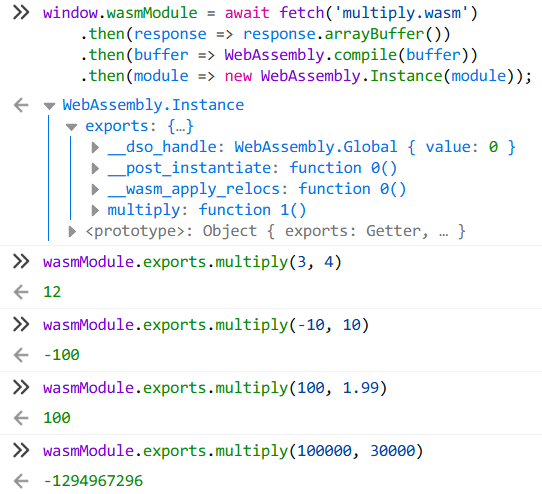Here is the corresponding “assembly code”, `\$multiply` is the above multiplication function.

 `````` 1 2 3 4 5 6 7 8 9 10 11 12 13 14 15 16 17 `````` ``````(module (type \$t0 (func)) (type \$t1 (func (param i32 i32) (result i32))) (func \$__wasm_apply_relocs (type \$t0) nop ) (func \$multiply (type \$t1) (param \$p0 i32) (param \$p1 i32) (result i32) local.get \$p0 local.get \$p1 i32.mul ) (global \$__dso_handle i32 (i32.const 0)) (export "__wasm_apply_relocs" (func \$__wasm_apply_relocs)) (export "multiply" (func \$multiply)) (export "__dso_handle" (global 0)) (export "__post_instantiate" (func \$__wasm_apply_relocs)) ) ``````

Using the VSCode extension WebAssembly you can display open wasm files in text format and convert between the two formats. between the two formats.

## Using external functions, memory and pointers

The next step is to do something that beginners of every programming language love to do: output a Hello world.

 ``````1 2 3 4 5 6 `````` ``````#include void hello_world() { puts("Hello world!"); puts("WebAssembly模块测试"); } ``````

Compile …… Wait a minute, since it is compiled to WebAssembly, where do I find the standard library `stdio.h` and this `puts`? Look at the text format code.

 `````` 1 2 3 4 5 6 7 8 9 10 11 12 13 14 15 16 17 18 19 20 21 22 23 24 25 26 27 28 `````` ``````(module (type \$t0 (func)) (type \$t1 (func (param i32) (result i32))) (import "env" "puts" (func \$env.puts (type \$t1))) (import "env" "__memory_base" (global \$env.__memory_base i32)) (import "env" "memory" (memory \$env.memory 0)) (func \$__wasm_apply_relocs (type \$t0) nop ) (func \$hello_world (type \$t0) (local \$l0 i32) global.get \$env.__memory_base local.tee \$l0 call \$env.puts drop local.get \$l0 i32.const 13 i32.add call \$env.puts drop ) (global \$__dso_handle i32 (i32.const 0)) (export "__wasm_apply_relocs" (func \$__wasm_apply_relocs)) (export "hello_world" (func \$hello_world)) (export "__dso_handle" (global 1)) (export "__post_instantiate" (func \$__wasm_apply_relocs)) (data \$d0 (global.get \$env.__memory_base) "Hello world!\00WebAssembly\e6\a8\a1\e5\9d\97\e6\b5\8b\e8\af\95\00") ) ``````

`puts` is something you need to import from JS. You can actually leave `#include <stdio.h>` out of the C code and just declare it with `int puts(const char *str);`.

The strings are all written into a single data segment (the Chinese part uses the same UTF-8 encoding as the source code). When loading the wasm module, you need to provide an area of memory to hold this data from an offset position.

Here we start by using `console.log` instead of `puts` and create a `WebAssembly.Memory` as a memory area, which is passed to the wasm module to be loaded via an object (i.e. `importObject` above).

 `````` 1 2 3 4 5 6 7 8 9 10 11 12 `````` ``````const wasmMemory = new WebAssembly.Memory({ initial: 1 }); const wasmBuffer = new Uint8Array(wasmMemory.buffer); const wasmModule = await fetch('hello-world.wasm') .then(response => response.arrayBuffer()) .then(buffer => WebAssembly.compile(buffer)) .then(module => new WebAssembly.Instance(module, { env: { puts: console.log, memory: wasmMemory, __memory_base: 0, } })); ``````

`WebAssembly.Memory` is a block of memory for wasm modules, allocated in 64 KB “pages” as a base unit, and can be dynamically expanded after creation. It can be read and written to in JS using `TypedArray`.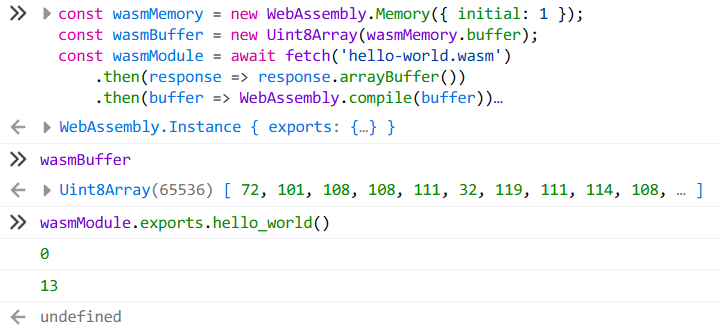The string is written to a location in memory starting at `__memory_base`, and as in C, the argument to the call to `puts` (actually `console.log`) is a pointer to the start of the string (the array index). If you implement a `puts` yourself, you can output the string to the console (or to the innerText of some DOM on the page).

By the way, there is a library called Locutus that tries to use JS to implement standard libraries for other languages, although there are not many implementations for C ……

For example, to implement `sprintf` or `printf`, see here.

 `````` 1 2 3 4 5 6 7 8 9 10 11 12 13 14 15 16 17 18 19 20 `````` ``````const wasmMemory = new WebAssembly.Memory({ initial: 1 }); const wasmBuffer = new Uint8Array(wasmMemory.buffer); const wasmModule = await fetch('hello-world.wasm') .then(response => response.arrayBuffer()) .then(buffer => WebAssembly.compile(buffer)) .then(module => new WebAssembly.Instance(module, { env: { // Decode the data between the start of the pointer and the next 0x00 in UTF-8, and then output it with console.log puts: ptr => console.log( (new TextDecoder).decode( wasmBuffer.slice( ptr, wasmBuffer.findIndex((e, i) => i >= ptr && !e) ) ) ), memory: wasmMemory, __memory_base: 0, } })); ``````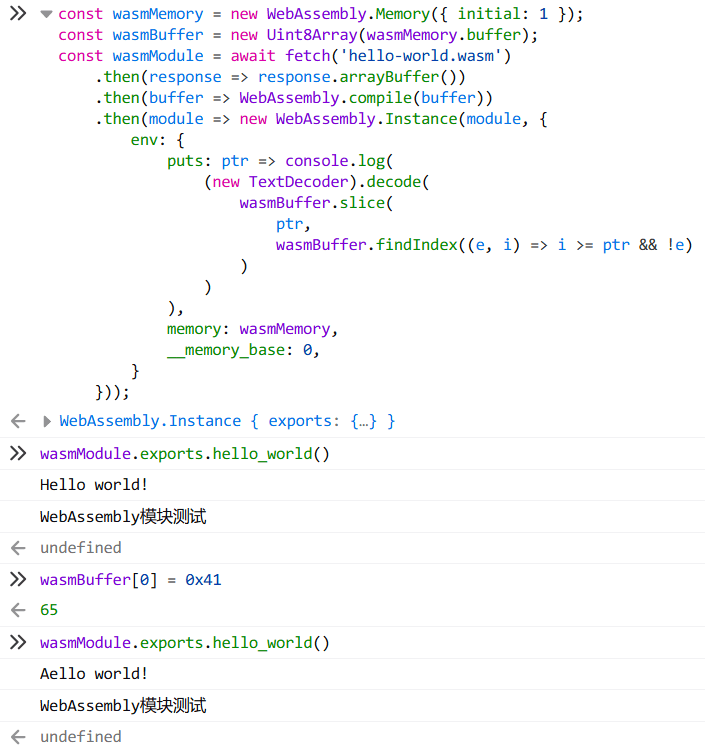Try to change the data of the first cell in memory to `0x41` (that is, the letter A), call the function again, and you will see that the output has changed accordingly. Of course, in practice, if you need to write data to memory, you have to reserve memory space according to `__memory_base` and the size of the data segment in your code to avoid overwriting the data used inside the wasm module.

If you need to use local variables in your code, common sense tells you that these variables are stored on the stack, and the compiled wasm will require the introduction of `__stack_pointer` to set the stack pointer.

 `````` 1 2 3 4 5 6 7 8 9 10 11 `````` ``````{ env: { __stack_pointer: new WebAssembly.Global( { mutable: true, value: 'i32', }, 0x1000, ), }, } ``````

Like the real CPU, the stack pointer extends to the lower address, so we get a simple process address space model like this: `| read-only data | <- stack | heap -> |`, which is starting to get a bit involved in operating system knowledge ……

Now that we mention the heap, can we use `malloc` and `free` to dynamically allocate memory on the heap? However, even without performance considerations, a full memory allocator is very complex and not usable in the case of such simple modules compiled as `SIDE_MODULE`, and will not be covered in depth here. The “Building an allocator” section of this article suggests a simple alternative: the allocated memory address is simply the starting address of the heap The allocated memory addresses are simply accumulated from the start of the heap, and as for `free`? Just leave it empty.

## An example of using WebAssembly to increase performance to 30x

The next step is to use a real-world example to demonstrate the superior performance of WebAssembly compared to JS for computationally intensive tasks. I tried to implement RC4 encryption and decryption algorithm in WebAssembly and JS respectively, why did I use RC4 as an example?

• Encryption and decryption involve a lot of computational operations
• RC4 is a short algorithm, and encryption and decryption are the same set of algorithms, so it is easy to implement.
• RC4 is a stream cipher, which does not require much plaintext and key length, and does not need to handle different working modes like packet ciphers, so it is easy to use
• has some practicality (although the security of RC4 is now more limited ……)

Here is the pseudo-code from Wikipedia.

 `````` 1 2 3 4 5 6 7 8 9 10 11 12 13 14 15 16 17 `````` ``````for i from 0 to 255 S[i] := i endfor j := 0 for( i=0 ; i<256 ; i++) j := (j + S[i] + key[i mod keylength]) % 256 swap values of S[i] and S[j] endfor i := 0 j := 0 while GeneratingOutput: i := (i + 1) mod 256 j := (j + S[i]) mod 256 swap values of S[i] and S[j] k := inputByte ^ S[(S[i] + S[j]) % 256] output K endwhile ``````

Follow the pseudo-code and use JS to implement it again (the implementation was verified to be completely correct, the verification process is omitted).

Implementation using JS.

 `````` 1 2 3 4 5 6 7 8 9 10 11 12 13 14 15 16 17 18 19 20 21 22 23 24 25 26 27 28 29 `````` ``````/** * @param {Uint8Array} key * @param {Uint8Array} input * @returns {Uint8Array} */ const jsRc4 = (key, input) => { let i = 0; let j = 0; const keyLength = key.length; const inputLength = input.length; const sbox = new Uint8Array(256); for (i = 0; i < 256; ++i) sbox[i] = i; for (i = 0; i < 256; ++i) { j = (j + sbox[i] + key[i % keyLength]) & 0xFF; [sbox[i], sbox[j]] = [sbox[j], sbox[i]]; } const output = new Uint8Array(inputLength); i = j = 0; for (let k = 0; k < inputLength; ++k) { i = (i + 1) & 0xFF; j = (j + sbox[i]) & 0xFF; [sbox[i], sbox[j]] = [sbox[j], sbox[i]]; output[k] = input[k] ^ sbox[(sbox[i] + sbox[j]) & 0xFF]; } return output; } ``````

Then ported to C.

Implementation using C language, and associated JS glue code

 `````` 1 2 3 4 5 6 7 8 9 10 11 12 13 14 15 16 17 18 19 20 21 22 23 24 25 26 27 `````` ``````static unsigned char sbox; void rc4(unsigned char *key, unsigned char *input, unsigned int keyLength, unsigned int inputLength) { unsigned short i = 0; unsigned char j = 0; unsigned char temp; for (i = 0; i < 256; ++i) sbox[i] = i; for (i = 0; i < 256; ++i) { // j = (j + sbox[i] + key[i % keyLength]) & 0xFF; j += sbox[i] + key[i % keyLength]; temp = sbox[i]; sbox[i] = sbox[j]; sbox[j] = temp; } i = j = 0; for (unsigned int k = 0; k < inputLength; ++k) { i = (i + 1) & 0xFF; // j = (j + sbox[i]) & 0xFF; j += sbox[i]; temp = sbox[i]; sbox[i] = sbox[j]; sbox[j] = temp; input[k] ^= sbox[(sbox[i] + sbox[j]) & 0xFF]; } } ``````

The test is to use KB random key to encrypt 4KB, 8KB ……128MB of the same random data using two implementations, and compare the execution times.

I realized after the test that I don’t need such a long key …… more than the first 256 bytes is meaningless, but it doesn’t affect the test results, so I won’t retest it!

Test Code.

 `````` 1 2 3 4 5 6 7 8 9 10 11 12 13 14 15 16 17 18 19 20 21 22 23 24 25 26 27 28 29 30 31 32 33 34 35 36 37 38 39 40 41 42 43 `````` ``````const results = []; let inputLength = 2048; for (let i = 0; i < 16; i++) { inputLength <<= 1; const rc4Key = new Uint8Array(1024).map(() => Math.random() * 256); const rc4Input = new Uint8Array(inputLength).map(() => Math.random() * 256); performance.mark('wasm-start'); const wasmEncrypted = wasmRc4(rc4Key, rc4Input); performance.mark('wasm-end'); performance.mark('js-start'); const jsEncrypted = jsRc4(rc4Key, rc4Input); performance.mark('js-end'); performance.measure('wasm', 'wasm-start', 'wasm-end'); performance.measure('js', 'js-start', 'js-end'); for (let j = 0; j < inputLength; j++) if (wasmEncrypted[j] !== jsEncrypted[j]) throw 'Not equal'; results.push({ length: inputLength, wasmTime: performance.getEntriesByName('wasm').duration, jsTime: performance.getEntriesByName('js').duration, }); performance.clearMarks(); performance.clearMeasures(); } results.push(results.reduce( (acc, cur) => { for (const key in acc) acc[key] += cur[key]; return acc; }, { length: 0, wasmTime: 0, jsTime: 0, } )); results.forEach(r => { r.wasmSpeed = r.length / r.wasmTime; r.jsSpeed = r.length / r.jsTime; r.ratio = r.wasmSpeed / r.jsSpeed; }); console.table(results); ``````

As for the test results, …… was first tested on my daily Firefox, and the performance was directly improved by 8x!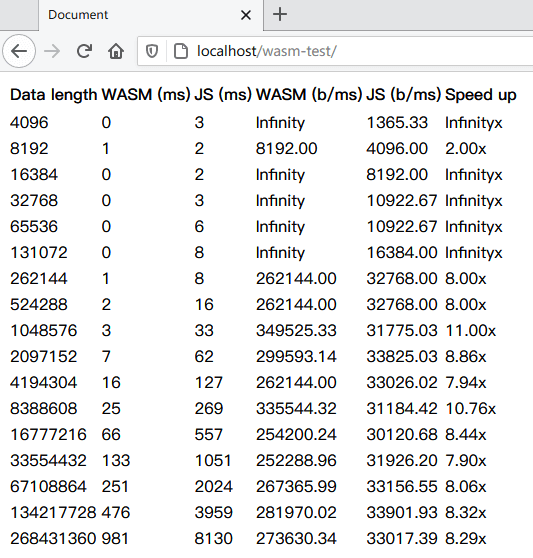Then there are the test results on Chrome. Even against Chrome, which uses the most powerful V8 engine, WebAssembly still has a performance advantage of 2x (although the speed is similar to that of Firefox ……)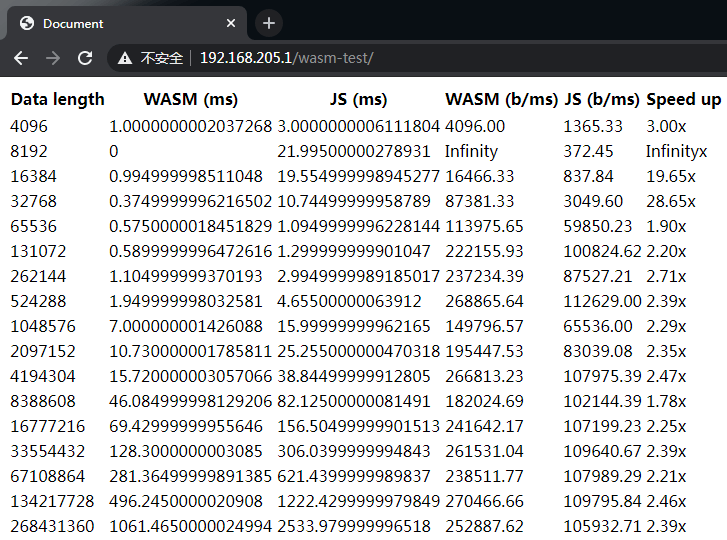As for the title, 30x…… is measured in the old Edge that has been abandoned, but the execution speed of both WebAssembly and JS is much worse than the other two.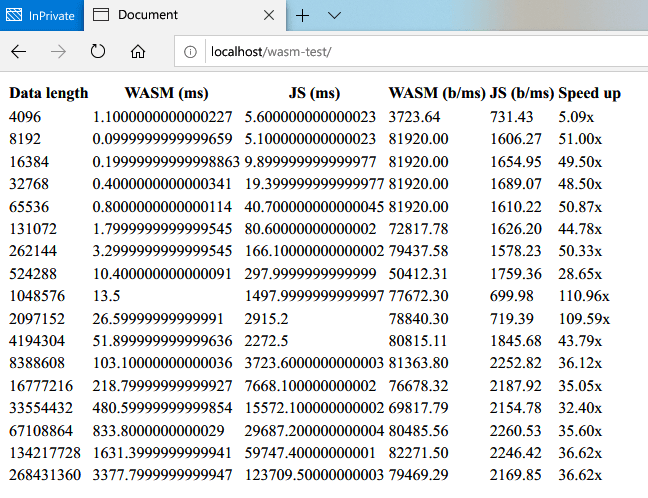You can also try it for yourself here ~ (wasm file is already embedded as a Data URL)

The conclusion is obvious, WebAssembly’s performance is much higher than JS. And if you don’t write the function name clearly, it’s hard to see the RC4 algorithm directly against its “assembly code”, so it might be a good idea to put some key operations into the wasm module to prevent the front-end code from being reversed?

Even if you’re not familiar with C and Emscripten’s set of tools, there are tools like Walt and AssemblyScript on GitHub assemblyscript) to write wasm modules directly using TypeScript syntax, so try that later!

## Extra: SIMD Support for WebAssembly

The WebAssembly standard has a proposal for a SIMD instruction set, and recently the major browsers and the JS engine for Node.js have finally implemented support for SIMD WebAssembly/simd/blob/main/proposals/simd/ImplementationStatus.md). In theory, using SIMD could further increase the execution speed of wasm (although this doesn’t seem to be the case in the simple tests I’ve done ……)

Referring to GoogleChromeLabs/wasm-feature-detect, SIMD support can be tested with the following code.

 ``````1 `````` ``````WebAssembly.validate(new Uint8Array([0, 97, 115, 109, 1, 0, 0, 0, 1, 5, 1, 96, 0, 1, 123, 3, 2, 1, 0, 10, 10, 1, 8, 0, 65, 0, 253, 15, 253, 98, 11])); ``````

According to the Emscripten documentation, compiling with the parameter `-msimd128` allows you to use SIMD optimization directly at compile time.